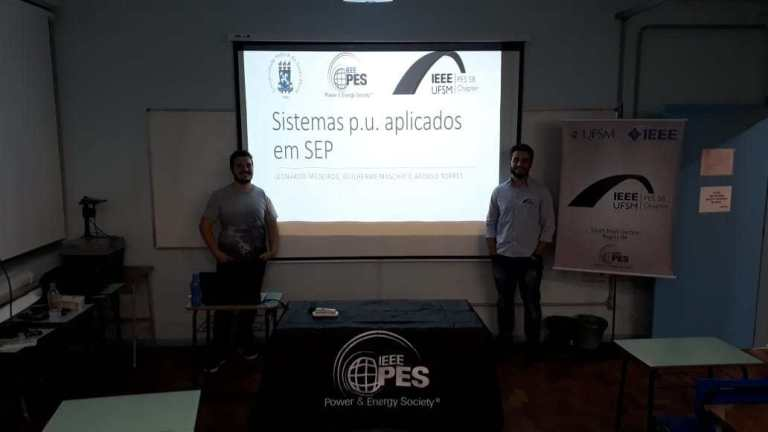• Sítios da UFSM
• Área restrita

Aviso de Conectividade Saber Mais

Início do conteúdo

# Per-unit Systems Applied to Power System

In the power systems analysis field of electrical engineering, a per-unit system is the expression of system quantities as fractions of a defined base unit quantity. Calculations are simplified because quantities expressed as per-unit do not change when they are referred from one side of a transformer to the other. This can be a pronounced advantage in power system analysis. Conversion of per-unit quantities to volts, ohms, or amperes requires a knowledge of the base that the per-unit quantities were referenced to. The per-unit system is used in power flow, short circuit evaluation, motor starting studies etc. The course Per-unit Systems Applied to Power System introduced and developed the p.u. systems applied to concepts and themes of power systems field to engineering students.

Objectives:

1. Introduce and develop the p.u. systems applied to power systems
2. Teach and review this classic methodology
3. Resolution of examples and academic exercises
4. Real examples

Courses Contents:

1. Power system introduction
2. Per-unit motivation
3. Per-unit introduction
4. Mono-phase system
5. Three-phase system
6. Base change
7. Transformers, motors, power factor
8. Complete analysisDivulgue este conteúdo:
https://ufsm.br/r-553-2991